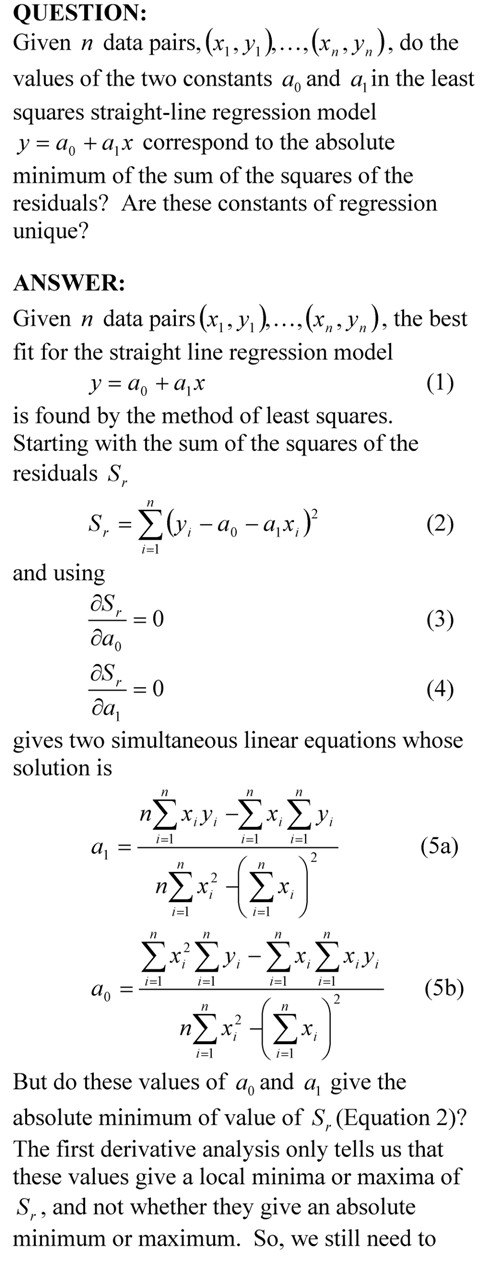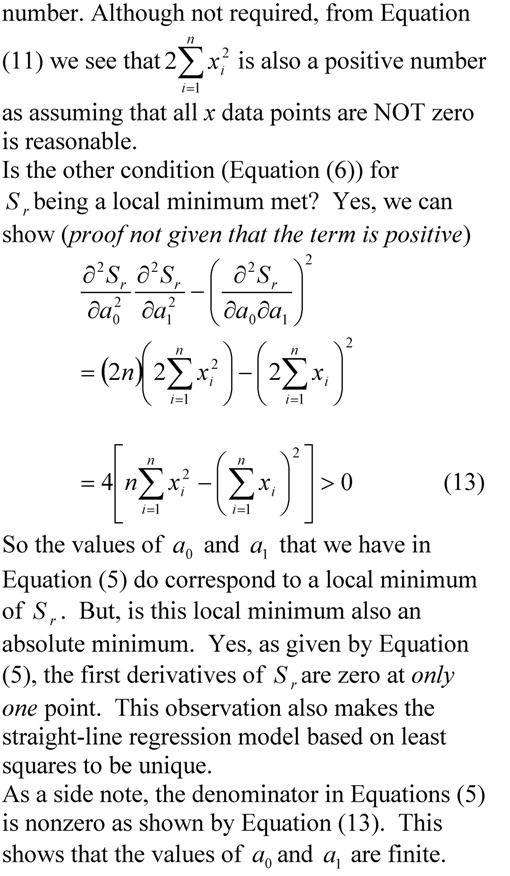## Sum of the residuals for the linear regression model is zero.

Prove that the sum of the residuals for the linear regression model is zero.________________________________________________

This post is brought to you by

• Holistic Numerical Methods Open Course Ware:
• the textbooks on
• the Massive Open Online Course (MOOCs) available at

## Proving the denominator of the linear regression formula for its constants is greater than zero.

In a previous blog, we wrote without proof that the denominator of the constants of the linear regression formulas are greater than zero.  In this blog, we show the proof.This post is brought to you by Holistic Numerical Methods: Numerical Methods for the STEM undergraduate at http://numericalmethods.eng.usf.edu, the textbook on Numerical Methods with Applications available from the lulu storefront, the textbook on Introduction to Programming Concepts Using MATLAB, and the YouTube video lectures available athttp://numericalmethods.eng.usf.edu/videos.  Subscribe to the blog via areader or email to stay updated with this blog. Let the information follow you.

## Prove that the least squares general straight-line model gives the absolute minimum of the sum of the squares of the residuals?

Many regression models when derived in books only show the first derivative test to find the formulas for the constants of a linear regression model.  Here we show a thoroughly explained derivation.___________________________________________

This post is brought to you by Holistic Numerical Methods: Numerical Methods for the STEM undergraduate at http://numericalmethods.eng.usf.edu, the textbook on Numerical Methods with Applications available from the lulu storefront, the textbook on Introduction to Programming Concepts Using MATLAB, and the YouTube video lectures available at http://numericalmethods.eng.usf.edu/videos.  Subscribe to the blog via a reader or email to stay updated with this blog. Let the information follow you.